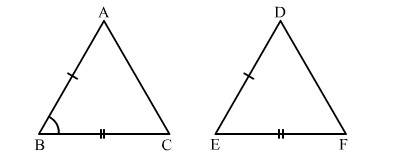# In ∆ABC and ∆DEF, it is given that AB = DE and BC = EF.

Question:

In ∆ABC and ∆DEF, it is given that AB DE and BC EF. In order that ∆ABC ≅ ∆DEF, we must have
(a) A = ∠D
(b) ∠B = ∠E
(c) ∠C = ∠F
(d) none of these

Solution:

(b) $\angle B=\angle E$In $\triangle A B C$ and $\triangle D E F$, we have :

AB = DE      (Given)
BC = EF       (Given)

In order that $\triangle A B C \cong D E F$, we must have $\angle B=\angle E$.